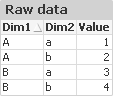# QlikView Creating Analytics

Discussion Board for collaboration related to Creating Analytics for QlikView.

HighlightedNot applicable

## Aggregation in dimension ignores previous dimensions

I have recently come across an issue with aggregations in chart dimensions that I have been able to resolve, but don't really understand the for.

My understanding of chart dimensions so far was that they are evaluated based on the restrictions of the preceding dimensions (so if the first dimension in on FieldA, then the second dimension will be calculated based only on those records with the respective FieldA value).

When using aggregation in a chart dimension (using aggr), the restrictions of previous dimensions seem to be ignored however if those dimensions are not included as aggregation dimensions for aggr.

Here is a short example that is also contained in the demo dashboard - it is based on the following source table:Now I create a chart with the following dimensions:

1. Dim1
2. Dim2
3. =aggr(sum(Value), Dim1)
4. =aggr(sum(Value), Dim1, Dim2)

And one very simple expression:

1. sum(Value)

My expectation would have been that aggregated columns return the same value (equal to the expression value), since there already is a restriction on Dim1 and Dim2 from the first two dimensions.

The actual output however is this:Can someone explain to me why it is necessary to include all the previous chart dimensions as dimensions in aggr in order to get the same result as the expression?

Tags (2)
1 Solution

Accepted SolutionsMVP

## Re: Aggregation in dimension ignores previous dimensions

Because the aggr() virtual table is solely built upon the information given in the aggr() function, the aggr() expression and the aggr() dimensions.

If the aggr() is used in an expression, the projection to outer chart dimension is performed in a second step,  taking the outer aggregation function into account.

2 RepliesMVP

## Re: Aggregation in dimension ignores previous dimensions

Because the aggr() virtual table is solely built upon the information given in the aggr() function, the aggr() expression and the aggr() dimensions.

If the aggr() is used in an expression, the projection to outer chart dimension is performed in a second step,  taking the outer aggregation function into account.Not applicable

## Re: Aggregation in dimension ignores previous dimensions

Wow - thanks swuehl for your insights on the inner workings of aggr()...within 10 minutes after posting the question